# Statistics - Knots (Cut points)

Knots are cutpoints that defines different regions (or partitions) for a variable.

In each regions, a fitting must occurs.

The definition of different regions is a way to stay local in the fitting process.

## Placement

One strategy is to decide K, the number of knots, and then place them at appropriate quantiles of the observed X.

Just spread them out uniformly, having roughly the same amount of data in each of the regions created by the knots.

The number of knots results in the number of parameters (see the formula)

The smoothing splines avoid the knot selection issues

Discover More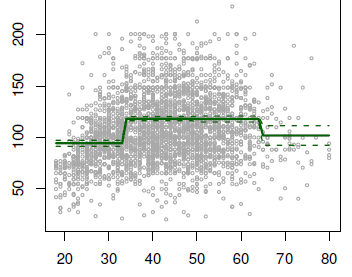Data Mining - Step Function (piecewise constants)

Step functions, are another way of fitting non-linearities. (especially popular in epidemiology and biostatistics) Continuous variable are cut into discrete sub-ranges and fit a constant model in...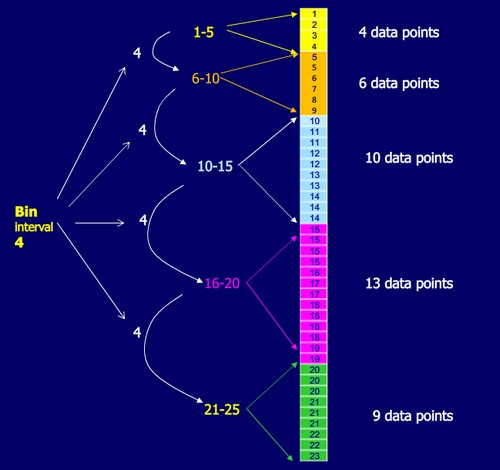Statistics - (Discretizing|binning) (bin)

Discretization is the process of transforming numeric variables into nominal variables called bin. The created variables are nominal but are ordered (which is a concept that you will not find in true...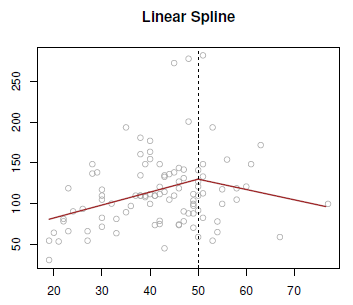Statistics - (Linear spline|Piecewise linear function)

A linear spline, or piecewise linear function has a degree zero and is: linear in the left and the right. forced to be continuous at the knot. Just like the global polynomials and the piecewise...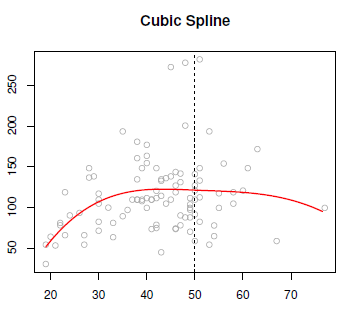Statistics - Cubic spline

Cubic splines is a step cubic piecewise polynomial in the left and the right and continuous at the knot. There's the following constraints: The first derivatives continues at the knot, and the...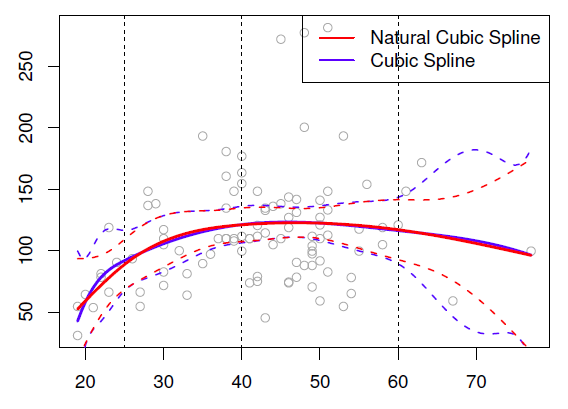Statistics - Natural (Cubic) Spline

A natural cubic spline is a cubic spline where two extra constraints have been added at the boundaries (on each end). The goal of this constraints is to avoid as for global cubic polynomial that the tail...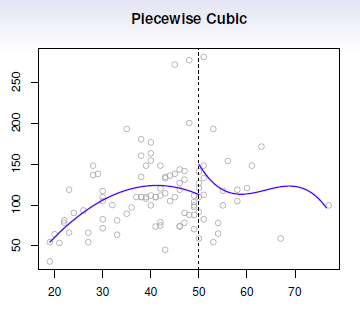Statistics - Piecewise polynomials

Piecewise polynomials generalize the idea of piecewise constants. The idea try to get rid of the global polynomial because it's global and not local. Instead of having asingle polynomial over the whole...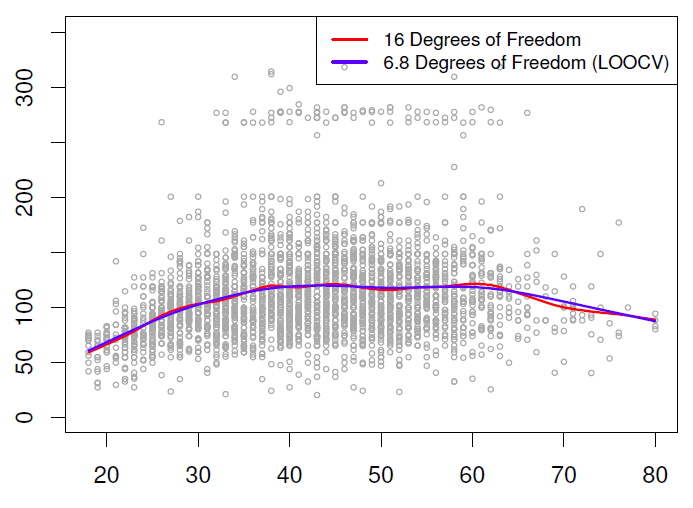Statistics - Smoothing (cubic) spline

A smoothing spline is a way of fitting splines without having to worry knots. They are a little bit more challenging mathematically as others splines and approaches the problem from a completely different...Statistics - Splines

A spline is a piecewise polynomial model: Splines have the “maximum” amount of continuity over all knots function.